Open in App
Not now

# Real Numbers

• Difficulty Level : Easy
• Last Updated : 18 Mar, 2021

In mathematics, a real number is a value of a continuous quantity that can represent a distance along a line. Real numbers include both rational and irrational numbers. Rational numbers such as integers (-5, 0, 9), fractions(1/2,7/8, 2.5), and irrational numbers such as √7, π, etc., are all real numbers.

Ever thought that we can count things, but how can we count. We can count by the use of numbers. But the number is also of many types some have negative values, some have positive values, some are very large, some are very small, some are in mathematical operations, so there are many types of numbers.

The number or the numeral system is the system of representing numbers. There are various types of number systems in maths like binary, decimal, etc. The Number system represents that how a number should be written.

Numbers are divided into the following types:

• Natural Numbers
• Whole Numbers
• Integers
• Rational Numbers
• Irrational Numbers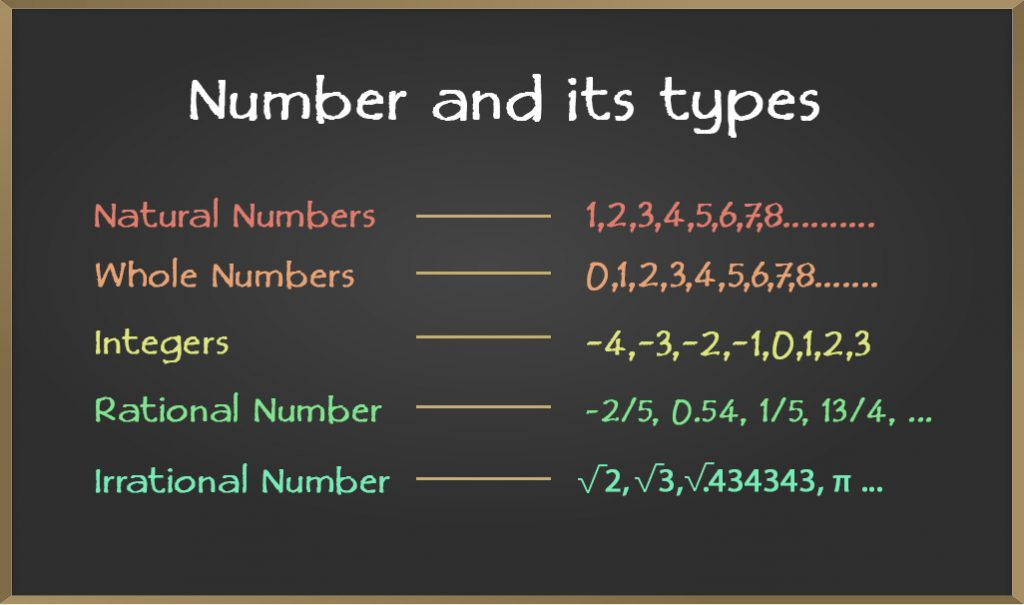### Natural Numbers

Natural numbers are those which are used by you in your daily life to count like 1, 2, 3 ….. These are positive numbers because we can’t count in negative terms.

Suppose you choose number from 1, 2, 3, 4, 5……and so on to infinity. These numbers are known as natural numbers. These natural numbers are denoted by the symbol N.

### Whole Numbers

Whole numbers are those numbers in which one number is added to Natural numbers. Adding 0 to Natural Numbers makes the series a set of Whole Numbers.

0, 1, 2, 3, 4, 5……and so on to infinity. These numbers are known as whole numbers. These whole numbers are denoted by the symbol W.

### Integers

All the number which have a complete value are known as integers and there are two type of integers first is negative and second is positive integers.

….-5, -4, -3, -2, -1, 0, 1, 2, 3, 4, 5……and so on to infinity. These numbers are known as integers and are denoted by the symbol Z.

### Rational Numbers

In mathematics, a rational number is a number that can be expressed as the fraction p/q of two integers, a numerator p, and a non-zero denominator q such as 2/7.

Example: 25 can be written as 25/1, so it’s a rational number.

### Irrational Numbers

Irrational numbers are those which can not be expressed in the form of p/q where p and q are both integers and q ≠ 0. In short, irrational numbers are real numbers that are not rational numbers.

Example: √3, √5, π, etc. These numbers are known as irrational numbers.

Question 1. Find three rational number between 6 and 7.

Three rational numbers between 6 and 7 are 13/2, 20/3 27/4.

Question 2. Can you identify following series 0, 1, 2, 3, 4, 5, 6, 7 ……?

This is a group of numbers representing whole numbers.

### Real Numbers Chart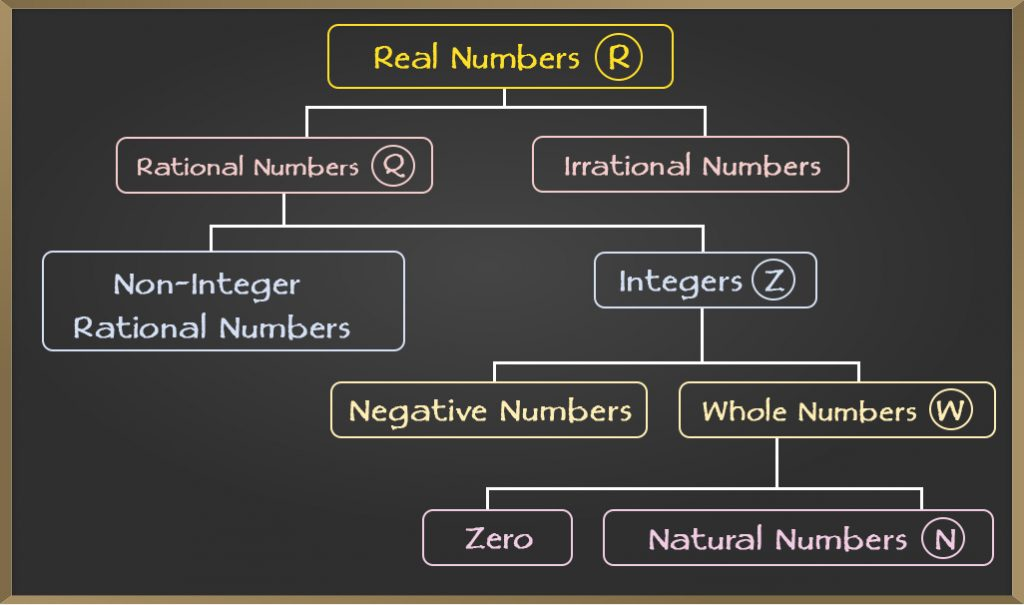### Representation of Numbers on the Number Line

A number line is a representation of Numbers with a fixed interval in between on a straight line. A Number line contains all the types of numbers like natural numbers, rationals, Integers, etc.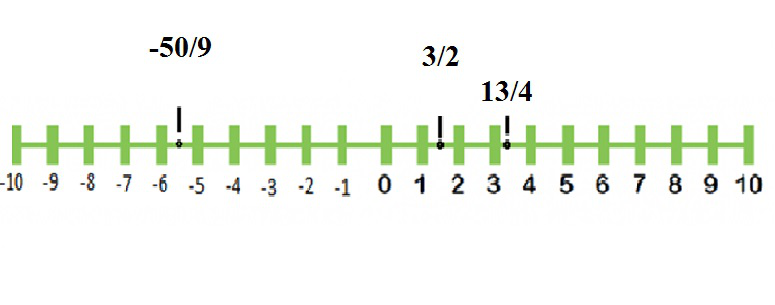As shown in the above number line 0 is present in the middle of the line. Positive integers are written on the right side of zero whereas negative integers are written on the left side of zero.

Rational numbers are written between the numbers they lie. For example, 3/2 equals to 1.5, so is noted between 1 and 2. It shows that the number 3/2 lies somewhere between 1 and 2.

Similarly, Number 13/4 lies between 3 and 4. So we noted it between 3 and 4. Number -50/9 lies between -5 and -6. So we noted it between -5 and -6 on the number line.

Question: Represent Following numbers on number line.

(i) 23/5

(ii) 6

(iii) -33/7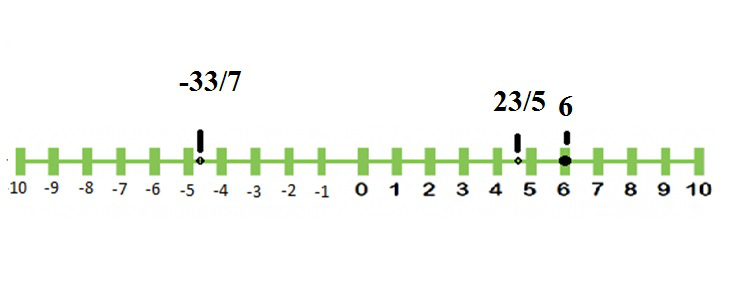### Decimal Expansion of a Real Number

The decimal expansion of a real number is its representation in base equals to 10 (i.e., in the decimal system). In this system, each “decimal place” consists of a digit from 0 to 9. These digits are arranged such that each digit is multiplied by a power of 10, decreasing from left to right.

Can we represent 13/4 in another form that can show its exact value on the number line?

The answer is yes. We can write it in decimals which gives its exact value. Let’s Expand 13/4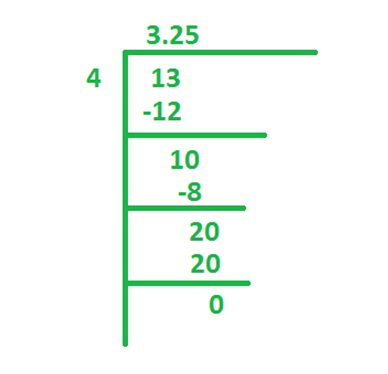So 13/4 can also be written as 3.25.

Now take another example. Let’s expand 1/3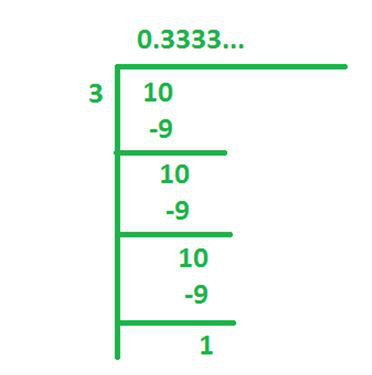So 1/3 can also be written as 0.3333…… We can also write it asSimilarly, 1/7 can be written as 0.142857142857142857… or. This can be defined as recurring decimals.

### Successive Magnification

The process of representation and visualization of real numbers on the number line through magnifying glass is known as successive magnification.

Let’s take an example of 3.25

We can tell that 3.25 definitely lies between 3 and 4. Can we tell where exactly it lies? Yes, we can do so using successive magnification.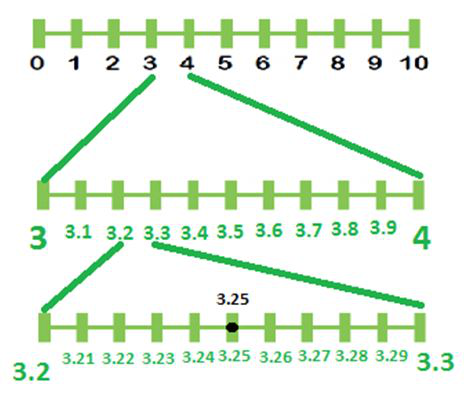In the first line, we see that 3.25 lies between 3 and 4. Now take it a step forward. Now we zoom between 3.2 and 3.3. Here we found that 3.25 lies between 3.2 and 3.3. So we represented 3.25 on the number line using successive magnification.

### Operations on Real Numbers

We know that we can perform mathematical operations on rational numbers. For example, we can add, divide, multiply and subtract the rational number with another number. As a result, we also get a rational number.

Similarly, we can perform mathematical operations on irrational numbers also, but the result can be rational or irrational.

### Sample Examples

Example 1: Add √3 and √5

Solution:

(√3 + √5)

Now answer is an irrational number.

Example 2: Multiply √3 and √3.

Solution:

√3 × √3 = 3

Now answer is a rational number.

So we can say that result of mathematical operations on irrational numbers can be rational or irrational.

Now add a rational number with an irrational number.

Example 3: Add 2 and √5

Solution:

(2 + √5)

Now answer is an irrational number.

Example 4: Simplify the expression: (2 + √3)(5 + √3)

Solution:

(2 + √3)(5 + √3)

= 10 + 2√3 + 5√3 + 3

= 13 + 7√3

Now answer is an irrational number.

My Personal Notes arrow_drop_up
Related Articles